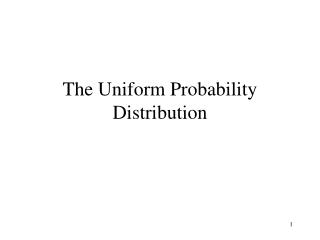Download PresentationThe Uniform Probability Distribution# The Uniform Probability Distribution - PowerPoint PPT Presentation

Download Presentation##### The Uniform Probability Distribution

Download Policy: Content on the Website is provided to you AS IS for your information and personal use and may not be sold / licensed / shared on other websites without getting consent from its author. While downloading, if for some reason you are not able to download a presentation, the publisher may have deleted the file from their server.

- - - - - - - - - - - - - - - - - - - - - - - - - - - E N D - - - - - - - - - - - - - - - - - - - - - - - - - - -
##### Presentation Transcript

1. The Uniform Probability Distribution

2. The height of the rectangle A rectangle The base of the rectangle Do you remember how to calculate the area of a rectangle from your earlier schooling? No problem, it is the base times the height! What if the base equals the height? We would have b times h or b times b (since b = h) or b2, where we say b squared. What do we call that special type of rectangle where the base equals the height? A circle, right?

3. I will come back to the rectangle, it will be important for what we will do. Let’s talk about a problem. Say a plane flying to the Big Apple from the old Second City takes anywhere from 120 to 140 minutes (from take off to touch down.) Say the distribution of flying time is uniform over the interval 120 to 140. This basically means the probability of each 1 minute interval between 120 and 140 has the same probability of occurring. IN other words, the plane has the same chance of landing in the 120 to 121 interval as the 121 to 122 interval and so on.

4. f(x) 1/20 x = minutes of flight time. 0 120 140 a b 1/(b – a)

5. On the previous screen you see a rectangle. It is on the interval 120 to 140 because this is the number of minutes a plane would take when flying to New York from Chicago. In general we say the interval is from a to b. Then the base of the rectangle is b – a = 140 –120 = 20 in our example. Now, here is an important point based on rules of probability. Remember that the sum of all the probabilities of the outcomes must sum to 1. Since we want the area to represent probability the area of the rectangle must equal =1. We do this by making the height 1/(b – a) = 1/(140 – 20) = 1/20. Base time height is then (20)(1/20) = 1 Now we can answer more questions dealing with the probability of an event when we have a uniform distribution.

6. What is the probability the plan will land between 122 and 124 minutes? Picture is on the next screen. We just want to find the area inside the rectangle between 122 and 124. The base is 124 – 122 = 2 and the height is 1/20, so we have a probability 2(1/20) = .1. You might see the same problem asked in the following way: P(122 <= x <= 124), or what is the probability x is greater than or equal to 122, but less than or equal to 124? Special case P(x = 125)? What is the probability the plane lands in 125 minutes? Well 125 – 125 = 0 and 0(1/20) = 0. Since probabilities are areas for continuous variable, the probability for any one value is zero. We can only do probabilities over an interval.

7. f(x) 1/20 x = minutes of flight time. 0 120 122 124 140 a b 1/(b – a)

8. In our example the mathematical expression for the probability density function is 1/20 for 120 <= x <= 140 f(x) = 0 elsewhere, and in general is 1/(b – a) for a <= x <= b f(x) = 0 elsewhere All this really means is that the height of the rectangle is just the inverse of the length of the rectangle.

9. The expected value for a uniform variable is (I do not have a proof here so hopefully you can accept this.) E(x) = (a + b)/2 and in our example equals (120 + 140)/2 = 130. The variance is Var(x) = (b – a)2/12 and in our example equals (140 – 120)2/12 = 400/12 = 33.33

10. Let’s do problem 6 page 246 The uniform distribution suggested by the problem looks like 8 11.975 12.1 .125

11. Part a is probability that we are between 12 and 12.05 We want this area and it is (12.05 – 12)8 = .05(8) = .4 8 12 12.05 11.975 12.1 .125

12. Part b is probability that we are above 12.02 We want this area and it is (12.1 – 12.02)8 = .08(8) = .64 8 12.02 11.975 12.1 .125

13. Part c is probability that we are above 12.02 or below 11.98 We want these areas and they add up to (12.1 – 12.02)8 + (11.98 – 11.975)8 = .08(8) + .005(8) = .64 + .04 = .68 This is also equal to 1 – [(12.02 – 11.98)8] = 1 - .32 = .68 8 11.98 12.02 11.975 12.1 .125